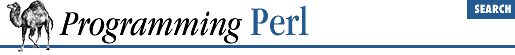home | O'Reilly's CD bookshelfs | FreeBSD | Linux | Cisco | Cisco Exam

#### 3.2.167 system

```system ```

LIST
```
```

This function executes any program on the system for you. It does exactly the same thing as ``` exec``` ``` LIST ``` except that it does a fork first, and then, after the exec, it waits for the exec'd program to complete. That is (in non-UNIX terms), it runs the program for you, and returns when it's done, unlike exec , which never returns (if it succeeds). Note that argument processing varies depending on the number of arguments, as described for exec . The return value is the exit status of the program as returned by the wait (2) call. To get the actual exit value, divide by 256. (The lower 8 bits are set if the process died from a signal.) See exec .

Because system and backticks block ``` SIGINT``` and ``` SIGQUIT``` , killing the program they're running with one of those signals doesn't actually interrupt your program.

```@args = ("command", "arg1", "arg2");
system(@args) == 0
or die "system @args failed: \$?"```

Here's a more elaborate example of analyzing the return value from system on a UNIX system to check for all possibilities, including for signals and coredumps.

```\$rc = 0xffff & system @args;
printf "system(%s) returned %#04x: ", "@args", \$rc;
if (\$rc == 0) {
print "ran with normal exit\n";
}
elsif (\$rc == 0xff00) {
print "command failed: \$!\n";
}
elsif ((\$rc & 0xff) == 0) {
\$rc >>= 8;
print "ran with non-zero exit status \$rc\n";
}
else {
print "ran with ";
if (\$rc &   0x80) {
\$rc &= ~0x80;
print "coredump from ";
}
print "signal \$rc\n"
}
\$ok = (\$rc == 0);```